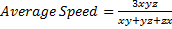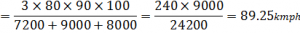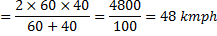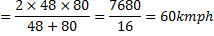# SBI CLERK PRELIMS – APTITUDE- 7

Dear Aspirants,

The SBI CLERK Prelims Exam 2018 is one of the important exam and we know you would be searching for the correct planner to devise your strategy. Since the exam pattern is changed , you would have been puzzled about how to study for the exam and make your preparations in the right way. Don’t fret and prepare as you wish but go in the right direction.

The Preliminary Examination of the SBI CLERK 2018 will happen in March/April , so you have to gear up your preparations in the right way to score more in the exams. So attend Daily Quizzes which will enrich your preparations and study and learn new topics which will help you for the exams.

Our Team will be providing the Daily Quizzes on various topics which were based on the previous exams and new pattern questions. This will help you to crack the exam and to achieve your goal. So attend and take the best result which could guide you to find the key of your dreams.SBI Clerk Prelims 2018 Study Planner Day – 7

Section : Quantitative Aptitude

Topic : Average – 1

Time: 20 Minutes

1. In a class of 52 students, the number of boys is two less than the number of girls. Average weight of the boys is 42 kg, While the average weight of all the 52 student is 40 kg. Approximately what is the average weight of the girls?

a) 41kg

b) 39kg

c) 40 kg

d) 38kg

e) 42kg

2. The average Income of P, Q, R, S and T is Rs 15000. The average income of P &Q is Rs 12000 and the average income of R&S is Rs 16000, find the average income of T.

a) Rs 19000

b) Rs 20000

c) Rs 23000

d) Rs 12000

e) none of these

3. The average number of coins with A, B, C and Dare 5. The average number of coins with D, E and F are 7. Find the number of coin with all the 6 people is 6.

a) 3

b) 5

c) 4

d) 7

e) 8

4. Samdha travels 1/3rd of distance from delhi to Jaipur at speed of 80km, nest 1/3rd distance at speed of 100kn. The average speed of Samdha for the entire journey is:

a) 90km

b) 89.25km

c) 90.16km

d) None of these

5. Gabbar Singh travelled from Ramgarh to Vijaygarh at speed of 60km and fromVijaygarh to laxmangarh at speed of 40km. then he returned back from laxmangarhto Ramgarh to meet Basanti, at speed of 80 km. if the distance between Ramgarh and Vijaygarh is same as distance between Vijaygarh and laxmangarh, then find the average speed of Gabbar Singh for the whole journey:

a) 48km

b) 60km

c) 56 km

d) None of these

e) 67km

6. The average income of all the employees of ABC Ltc is Rs 12000 in 1999. In the year 2000, the company gave a bonus of Rs 2000 to each of the employees,then the new average is

a) Rs 12000

b) Rs 1400

c) Rs 14000

d) None of these

e) Rs 120000

7. The average expenditure of P, Q and R is Rs 5000 per month. Also, the average expenditure of Q, R and S is Rs 7000 per month. If the average expenditure of S is thrice of that of P then the average expenditure of Q and R is:

a) Rs 6000

b) Rs 3000

c) Rs 5000

d) Rs 4000

e) none of these

8. The average age of 8 person is increased by 2 years when one of them, whose age is 24 years id replaced by a new person. The age of new person is

a) 42 years

b) 40years

c) 38years

d) 45 years

e) None of these

9. The average age of five officers in a department is 32 years. If the age of their supervisor is added, the average age increased by 1. What is the supervisor’s age?

a) 32years

b) 48years

c) 38years

d) 42 years

e) None of these

10. In a one-day cricket match the captain of one of the teams scored 30 runs more than the average runs scored by the remaining six batsmen of that team who batted in the match. If the total runs scored by all the batsman of that were 310, how many runs did the captain score?

a) 60

b) 70

c) 50

d) Cannot be determined

e) None of these

11) Average score of Rahul, Manish and Suresh is 63. Rahul’s score is 15 less than Ajay and 10 more than Manish. If Ajay scored 30 marks more than the average score of Rahul, Manish and Suresh, what is the sum of Manish’s and Suresh’s scores?

(a) 120

(b) 111

(c) 117

(d) Cannot be determined

(e)None of these

12) The sum of 8 consecutive odd numbers is 656. Also average of four consecutive even numbers is 87. What is the sum of the smallest odd number and second largest even number?

(a) 165

(b) 175

(c) 163

(d) Cannot be determined

(e) None of these

13) The average marks in English subject of a class of 24 students are 56. If the marks of three students were misread as 44, 45 and 61 of the actual marks 48, 59 and 67 respectively, then what would be the correct average?

(a) 56

(b) 55

(c) 57.5

(d) 58.5

(e) None of these

14) The average age of some males and 15 females is 18 years. The sum of the ages of 15 females is 240 years and average age of males is 20 years. Find the number of males.

(a) 8

(b) 7

(c) 10

(d) 15

(e) None of these

15) The sum of the present ages of P and Q is 25 years more than the age of R. The present age of is 5 years more than that of R. Find the present age of P.

(a) 20 years

(b) 25 years

(c) 21 years

(d) 22 years

(e) None of these

16) The average of four consecutive even numbers P, Q, R and S respectively (in increasing order) is 51. What is the product of P and R?

(a) 2592

(b) 2400

(c) 2600

(d) 2808

(e) None of these

17) The average height of 27 per-sons was recorded as 162 cm. If the height of Shreya was deleted from the observation, the average height reduced by I cm. What was Shreya’s height?

(a) 184 cm.

(b) 226 cm.

(c) 179 cm.

(d) 186 cm.

(e) None of these

18) The average of the 9 consecutive positive integers is 63. The product of the largest and smallest integer is

(a) 3935

(b) 3953

(c) 3853

(d) 3835

(e) 3635

19) The present age of Romila is one fourth of that of her father. After 6 years the father’s age will be twice the age of Kapil. If Kapil celebrated fifth birth day 8 years ago, what is Romila’s Present age?

(a) 7 years

(b) 7.5 years

(c) 8 years

(d) 8.5 years

(e) None of these

20) The average height of 16 students is 142 cm. If the height of the teacher is included, the average height increases by 1 cm. The height of the teacher is

(a) 156 cm

(b) 159 cm

(c) 158 cm

(d) 157 cm

(e) 159.5 cm

Explanation for the Above Questions :

1.d) 38kg

Explanation:

let the number of boys=x; and

number of girls = y

Now, (y-2)=x and x+y=52

y-2+y=52

2y=54

Y=27

X=25

Let the average weight of the girls be ‘a’.

Or 27×a=(52×40)-(25×42)

Or 27×a=2080-1050

a =1030/27

=38.148

=38

1. a) Rs 19000

Explanation:

sum of the incomes of P, Q, R, S and T=15000× 5=75000

sum of the incomes of P, Q=2×12000=24000

sum of the incomes of R& S=2×16000=32000

Average income of T

= (P+Q+ R+S+T)-(P+Q)-(R+S)

= 75000-24000-32000=19000

1. b) 5

Explanation:

Total number of coins with A, B,C,& D=5×4=20

Total number of coins with D,E&F=3×7=21

Total number of coins with A, B, C, D, E & F=6×6=36

Number of coins with D=(A+B+C+D)+(D+E+F)-(A+B+C+D+E+F)

=20+21-36=5

1. b) 89.25km

Explanation:

Since distance travelled at different speed is same, we can use the short cut

Since distance travelled at different speed is same, we can use the short cut1. b) 60km

Explanation:

The average speed of gabbarsingh from Ramgarh to Laxmangath[Because distance from Ramgarh to Vijaygarh and from Vijaygarh to Laxmangathis same].

He is travel from Laxmangath  toRamgarh at speed of 80km

The average speed of entire journey1. c) Rs 14000

Explanation:

Since all the employees are given a bonus of Rs 2000, hence the average would also increase by Rs 2000.

1. a) Rs 6000

Explanation:

Total expenditure of P, Q & R=3×5000=15000

Total expenditure of Q, R & S =3×7000=21000

S-P=21000-15000=6000

Also, we are given S=3P. so 3P-P =6000 & P=3000

Total expenditure of Q & R=15000-3000=12000

Average expenditure of Q& R=12000/2 =6000

1. b) 40years

Explanation:

The average age of 8 person is increased by 2i.e sum of the age would increase by 16. Age of the new person would be 16 years more than age of the person replaced, so age3 of new person =24+16=40 years

1. c) 38years

Explanation:

The new average is increased by 1 year hence sum of the age would have increased by 6years. Age of supervisor =32+6=38 years

1. b) 70

Explanation:

Let the average runs scored by 6 batman =x

Total runs scored by them =6x

Then, 6x+x+30=310

7x=280

X=40

Score of captain=40+30=70

1. (b) 111

Explanation:

Ajay’s score = 63 + 30 = 93

Rahul’s score = 93 – 15 = 78

Sum of Manish’s and Suresh’s scores

= 3 x 63 – 78 = 189 – 78 = 111

1. (c) 163

Explanation:

Average of 8 consecutive odd numbers = (656/8) 82

Fourth number = 82 – 1 = 81

First number = 75

Average of 4 even numbers = 87

Second even number = 87 – 1 =86

Second largest even number = 88

Required sum = 75 + 88 = 163

1. (e) None of these

Explanation:

Difference = 48 + 59 + 67 44 – 45 – 61 =24

Correct average

= 56 + (24+24) =27

1. (d) 15

Explanation:

Let the number of males be x.

18x + 270 = 20x + 240

20x – 18x = 270 – 240

2x = 30

x=30/2=15

1. (a) 20 years

Explanation:

P + Q = R + 25 … (i)

Q = R + 5 …….. (ii)

Subtracting equation (ii) from equation (i),

P+Q-Q=R+ 25 – R – 5

P = 20 years

1. (e) None of these

Explanation:

x+x+2+x+4+x+6

= 4 x 51

4x + 12 = 204

4x = 204 – 12 = 192

x =  192/4  = 48 = P

R = 48 + 4 = 52

P x R = 48 x 52 = 2496

1. (e) None of these

Explanation:

Tricky approach

Height of Shreyas

= 162+26

= 188 cm

1. (b) 3953

Explanation:

Average of 9 consecutive positive integers = 63

Fifth number = 63

Smallest number = 59

Largest number = 67

Required product 47= 59 x 67 = 3953

1. (b) 7.5 years

Explanation:

Kapil’s present age  = 12 years

After 6 years, Kapil’s age  = 18 years

Father’s present age =36-6

= 30 years

Romila’s present age

(¼)  ×30=7.5years

1. (b) 159 cm

Explanation:

Tricky approach

Height of teacher = (142 + 17) cm

= 159 cm

Aspirants can also check the other topics from the SBI CLERK STUDY PLANNER from the link given below. DO bookmark the given link as all the posts will be updated in that page and aspirants can check the daily updates from this page or from the Homepage of Bankersdaily.